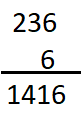# McGraw Hill Math Grade 4 Chapter 5 Lesson 2 Answer Key Multiplying a Three-Digit Number

Practice the questions of McGraw Hill Math Grade 4 Answer Key PDF Chapter 5 Lesson 2 Multiplying a Three-Digit Number to secure good marks & knowledge in the exams.

## McGraw-Hill Math Grade 4 Answer Key Chapter 5 Lesson 2 Multiplying a Three-Digit Number

Multiply

Find each product.

Question 1.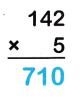142 x 5 = 710
Explanation:
The product of 142 and 5 is 710
take the carried value and place it in the column to the left.
So if you were multiplying numbers in the tens column and you needed to carry a number,
you would place that “number” in the hundreds column.

Question 2.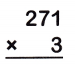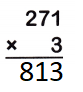Explanation:
The product of 271 and 3 is 813
take the carried value and place it in the column to the left.
So if you were multiplying numbers in the tens column and you needed to carry a number,
you would place that “number” in the hundreds column.

Question 3.Explanation:
The product of 309 and 8 is 2472
take the carried value and place it in the column to the left.
So if you were multiplying numbers in the tens column and you needed to carry a number,
you would place that “number” in the hundreds column.

Question 4.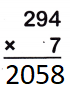Explanation:
The product of 294 and 7 is 2058
take the carried value and place it in the column to the left.
So if you were multiplying numbers in the tens column and you needed to carry a number,
you would place that “number” in the hundreds column.

Question 5.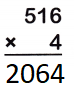Explanation:
The product of 516 and 4 is 2064
take the carried value and place it in the column to the left.
So if you were multiplying numbers in the tens column and you needed to carry a number,
you would place that “number” in the hundreds column.

Question 6.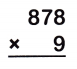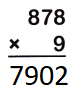Explanation:
The product of  878 and 9 is 7902
take the carried value and place it in the column to the left.
So if you were multiplying numbers in the tens column and you needed to carry a number,
you would place that “number” in the hundreds column.

Question 7.
A giant panda weighs 236 pounds. An Arabian camel weighs 6 times as much as the panda. How much does the Arabian camel weigh?